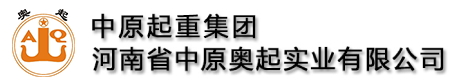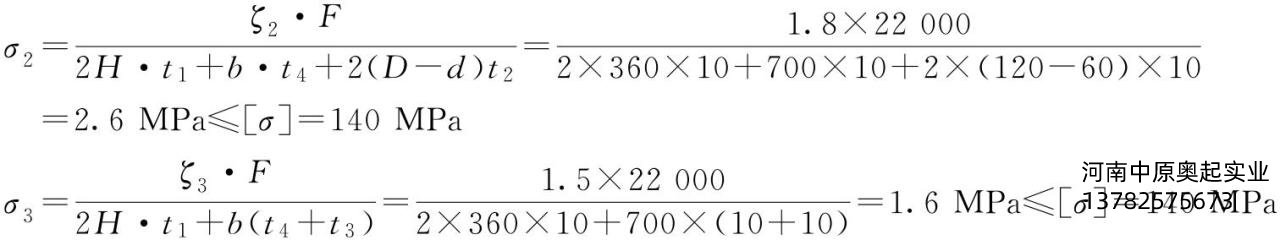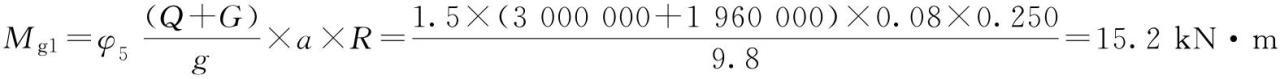全国统一服务热线:13782575673

# 龙门吊大车端梁的连接梁设计计算

### 来源：未知   发布时间：2021-03-18 14:46  点击量：

根据前面的计算和连接梁的设计可得以下参数(图4-58)。

t1=10mm;t2=10mm;t3=10mm;t4=10mm;b=700mm;h=320mm;H=360mm;F=22000N;d=60mm;D=120mm;ζ1取2.8;ζ2取1.8;ζ3取1.5;ζ4取1.8;m=60mm;￼。连接轴符合要求。连接梁符合要求。

(4)大车运行机构设计计算

①大车“三合一”减速器选型计算

a.大车摩擦阻力计算b.大车坡道阻力的计算

坡度角αsinα=0.001

坡道阻力Fp=(Q主+G)×sinα=(3000000+1960000)×0.001=4960N

c.大车运行静阻力的计算

Fj=Fm+Fp=42400+4960=47360N

d.大车运行静功率计算PN=kd·Pj=1.2×8.4=10.1kW

e.大车运行静阻力矩的计算

MP1=Fj×R=47360×0.250=11.8kN·m

式中　R——大车车轮的半径(m)，R=D/2。

大车满载直线运行产生的惯性力矩：加速度取0.08MH=MP1+Mg1=11.8+15.2=27kN·m

大车为四角驱动型式，使用四个减速器驱动，1个齿轮箱应承载MH/4=6.750kN·m。

Na=V/3.14×D=25.5

f.大车减速器选型

选取恒星科技国内品牌“三合一”K系列减速器：功率11kW;fb=2.0;i=54.99;n=27;Ma=3950N·m。

Ma×fb=3950×2.0=7900N·m>MH/4=6750N·m。

所选减速器符合要求。

②大车轮压计算

根据本例前面计算P大1/2=P0max=9.69×105/2=484.5kN;取Pmax=490kN。

③大车车轮设计计算

参考同类低净空起重机，初选大车车轮直径D=500mm，Pmin取P2min/2和P2min/2的小值，Pmin=147.5/2=73.75(kN);Pmax=490kN。大车轨道选QU100，车轮有效接触宽度￼车轮材料选DG42CrMo取σb=1080N/mm2，许用轮压比k=8.5。

允许轮压PL=kDlC=8.5×500×89.33×1.03×1=391.0kN。

疲劳强度校核：Pmean≤PL满足要求。

静强度校核：490(kN)=Pmax≤1.9kDl=1.9×8.5×500×89.33=721.3kN

车轮满足要求。

④大车缓冲器选型计算选某国内品牌聚氨酯缓冲器：YK-JHQ-C-20，缓冲容量为25.7kJ，符合要求。
文章来源：http://www.hnzyaq.com/gsxw/539.html

关键词：龙门吊